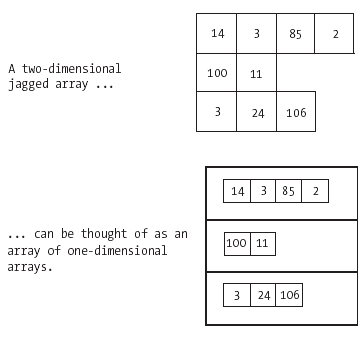FREE BOOK

# Chapter 6: Collections of Objects

Posted by Apress | C# Language December 16, 2008
The properties and behaviors of some common collection types,How collections enable us to model very sophisticated real-world concepts or situations,How we can define our own collection types

Multidimensional Arrays

So far we've been discussing one-dimensional arrays. It's also possible to declare and use arrays of two or more dimensions. There are two types of multidimensional arrays-rectangular and jagged.

Rectangular Arrays

A rectangular array is one in which every row has the same number of columns:

 3 2 37 8 4 7 4 9 0 3 1 11 99 13 5

This represents a three-row by five-column two-dimensional rectangular integer array.

The syntax for declaring and instantiating a two-dimensional rectangular array is as follows:

ArrayType[,] arrayName = new ArrayType[numRows, numCols];

For example:

double[,] values = new double[3, 5]; // three rows of five columns each

We place one comma between the brackets on the left-hand side to signal that the array will have two dimensions, and must then specify the size of each of the two dimensions, separated by a comma, on the right-hand side. For a three-dimensional rectangular array, we would use two commas between the brackets on the left-hand
side, and would specify the sizes of three dimensions on the right-hand side:

ArrayType[,,] arrayName = new ArrayType[dim1, dim2, dim3];

and so forth.

To access the elements of a multidimensional rectangular array, we use indexes, but now must specify an index for each dimension of the element to be accessed. For example, consider the following code snippet, in which we instantiate a two-dimensional array of type double:

double[,] data = new double[2, 3];
data[0, 1] = 23.4; // insert value into the FIRST row, SECOND column
// details omitted ...
double temp = data[1, 2]; // retrieve value from the SECOND row, THIRD column

The array has two rows and three columns. In the second line of code, the element in the first row and second column ([0, 1]-remember that we start counting from 0) of the array is assigned the value 23.4. In the third line, we're accessing the value of the element in the second row and third column of the array, and assigning that value to a double variable named temp.

The elements of a multidimensional rectangular array can be initialized at the time that the array is declared by placing the initial values in braces { ... }, with one set of braces for each dimension of the array. For example, the following syntax would create and initialize a two-row, three-column array of integer values:

int[,] data = { {7, 22, 3},
{48, 5, 10} };

Jagged Arrays

A jagged array is one where each row can have a different number of entries:

 14 3 85 2 100 11 3 24 106

This represents a three-row, two-dimensional jagged integer array. The syntax for declaring a jagged multidimensional array is different from that of a rectangular array.

• A separate set of empty braces is provided in the declaration for every dimension in the array: for a two-dimensional jagged array, we use two sets of braces; for a three-dimensional jagged array, we use three; etc. For example, the following syntax would declare a two dimensional jagged string array:

string[][] names; // note two sets of empty braces

• In the array instantiation statement, we only specify the size of the first dimension of such an array. For example, the following syntax would instantiate a two-dimensional jagged string array with three rows, allowing for a variable number of columns per row:

string[][] names = new string[];

In effect, when we create a two-dimensional jagged array, we have in essence created an array of one-dimensional arrays of varying sizes, as illustrated in Figure 6-5.Figure 6-5. A jagged two-dimensional array as an array of one-dimensional arrays

The next step in the process is to initialize the length of each row in the array; for example:

names = new string; // first row has 4 columns (numbered 0 ... 3)
names = new string; // second row has 2 columns (numbered 0 ... 1)
names = new string; // third row has 3 columns (numbered 0 ... 2)

To access the elements of a multidimensional jagged array, we use indexes with a separate set of brackets to specify each dimension. For example, to assign a value to the element in the second row and second column of the names array, we'd use the following syntax:

names = "Mel";

It's also possible to initialize the elements of a multidimensional jagged array when the array is first declared, but the syntax is a bit complicated. The elements of every one-dimensional array within the multidimensional array can be initialized by placing the initial values inside braces when the one-dimensional array is declared. The new keyword must be used, and the array type must also be specified. For example, the following code creates and initializes a two-dimensional jagged integer array that has three columns in its first row and four columns in the second row:

int[][] data = new int[];
data = new int[] { 17, 3, 24 };
data = new int[] { 6, 37, 108, 99 };